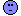## Danish ultra-conservatism [Power / Sample Size]

Dear Detlew,

» Side effect 2:
» The Danish are ultra-conservative!

Confirmed. Try this one:

library(PowerTOST) dk <- function(CV, n, lower, upper, theta0) { # Danish power         p0 <- power.TOST(CV=CV, n=n, theta0=theta0,                          theta1=lower, theta2=upper)         p1 <- power.TOST(CV=CV, n=n, theta0=theta0,                          theta1=lower, theta2=1)         p2 <- power.TOST(CV=CV, n=n, theta0=theta0,                          theta1=1, theta2=upper)         p0-p1-p2       } of <- function(x) { # objective function         (dk(CV=CV, n=n, lower=lower, upper=upper, theta0=x)-target)^2       } lower  <- 0.9 # NTID-range upper  <- 1/lower CV     <- 0.1 target <- 0.8 theta0 <- 0.9 n      <- 22 # fixed theta0.dk <- optimize(of, interval=c(lower, 1), tol=1e-12)\$minimum power  <- dk(CV=CV, n=n, lower=lower, upper=upper, theta0=theta0.dk) pwr.1  <- dk(CV=CV, n=n, lower=lower, upper=upper, theta0=theta0) GMR    <- seq(lower, upper, length.out=101) pwr    <- vector() for (j in seq_along(GMR)) {   pwr[j] <- dk(CV=CV, n=n, lower=lower, upper=upper, theta0=GMR[j]) } plot(GMR, pwr, log="x", ylim=c(0, 1), type="l", lwd=2, col="blue",      las=1, ylab="power with a Danish twist", cex.main=1,      main=paste0("theta0 = ", theta0, " (n = ", n, ")")) grid() abline(h=target) abline(v=c(theta0, 1/theta0), lty=3, col="red") abline(v=c(theta0.dk, 1/theta0.dk), lty=2, col="blue") arrows(theta0, 0, theta0.dk, 0, length=0.1, angle=25,        code=2, lwd=2, col="red") arrows(1/theta0, 0, 1/theta0.dk, 0, length=0.1, angle=25,        code=2, lwd=2, col="red") BE     <- sprintf("%s %.4f%s%.4f", "BE-limits:", lower, "\u2026", upper) pwr    <- sprintf("%s %.4g", "Power (theta0):", pwr.1) op     <- par(no.readonly=TRUE) par(family="mono") if (round(power, 6) >= target) {   legend("topright", bg="white", cex=0.9, x.intersp=0,          legend=c(BE, sprintf("%s %.4f%s%.4f",                               "GMR-range:", theta0.dk, "\u2026", 1/theta0.dk),                   pwr)) } else {   legend("topright", bg="white", cex=0.9, x.intersp=0,          legend=c(BE, sprintf("%s %.4f", "Achievable power:", power), pwr)) } par(op) 

It is courageous to assume theta0 0.9 for a NTID (sampleN.TOST() would tell you to get lost). If you believe in 0.95, n would be 44. To get 80% power the GMR-range is 0.9834…1.0169. Power for 0.95 is only 0.2333. Would it be ethical to perform the study?

»
Something wrong with the forum? It kicks me out nearly all the time.

You are not alone.See this post.

Dif-tor heh smusma 🖖
Helmut SchützThe quality of responses received is directly proportional to the quality of the question asked. 🚮
Science QuotesIng. Helmut Schütz